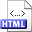Bucketing and filtering in computational geometry Author: Jyrki Katajainen Publication: Ph.D. Thesis, Department of Computer Science, University of Turku (1987), vi+120 pp. Abstract: This thesis summarizes the work on computational geometry which I have done during the last five years at the University of Turku. The computational complexity of three geometrical proximity problems is examined: How to construct a minimum spanning tree, the relative neighbourhood graph, and the Delaunay diagram (a Delaunay triangulation) for a finite set of points in a two-dimensional coordinate space with the Lp metric as a distance measure.   The main contributions of this work are: A simple expected-time optimal algorithm for relative neighbourhood graphs. The algorithm works for any Lp metric, 1 ≤ p ≤ ∞. Similar ideas can be used to obtain a simple algorithm for minimum spanning trees, as well as for Delaunay diagrams (triangulations).A simple expected-time and worst-case time optimal algorithm for Delaunay diagrams (triangulations). The algorithm works for any Lp metric, 1 < p < ∞.A worst-case optimal algorithm for relative neighbourhood graphs in L1 and L∞ metrics.   To some extent, the minimum spanning tree and relative neighbourhood graph problems are also considered in a multidimensional space. Two methodologies, bucketing and filtering, are extensively used in developing the algorithms. Most of the algorithms proposed are implemented, and compared experimentally with existing algorithms. Related:HTML (Journal article)HTML (Journal article)HTML (Journal article)HTML (Journal article)HTML (Journal article)HTML (Journal article) BibLATEX: ```@thesis{PhDThesis1987T, author = {Jyrki Katajainen}, title = {Bucketing and filtering in computational geometry}, type = {Ph.D. Thesis}, institution = {Department of Computer Science, University of Turku}, year = {1987}, pagetotal = {vi+120}, } ```